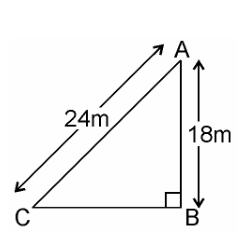Guru

# A guy wire attached to a vertical pole of height 18 m is 24 m long and has a stake attached to the other end. How far from the base of the pole should the stake be driven so that the wire will be taut? Q.10

• 0

The best way to solve the problem of class 10th ncert book of triangles chapter of exercise 6.5 of question no.10, how i solve this tough question in easy way, A guy wire attached to a vertical pole of height 18 m is 24 m long and has a stake attached to the other end. How far from the base of the pole should the stake be driven so that the wire will be taut?

Share

1. Given, a guy wire attached to a vertical pole of height 18 m is 24 m long and has a stake attached to the other end.Let AB be the pole and AC be the wire.

By Pythagoras theorem,

AC2 = AB2 + BC2

242 = 182 + BC2

BC= 576 – 324

BC= 252

BC = 6√7m

Therefore, the distance from the base is 6√7m.

• 0